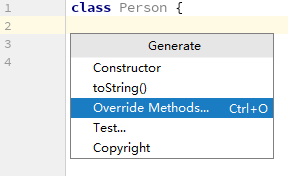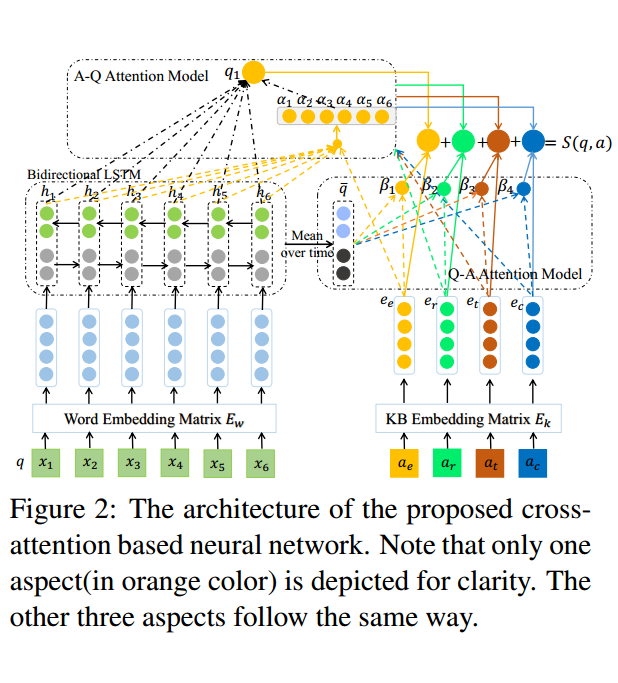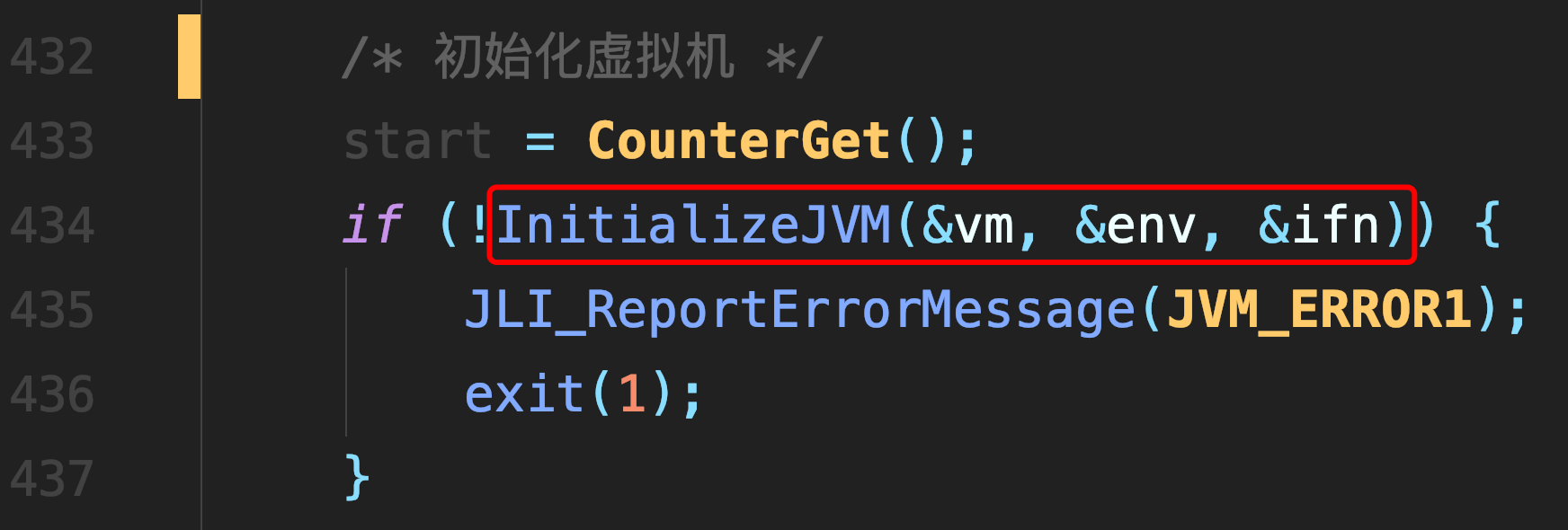# single-number

### 题目描述

Given an array of integers, every element appears three times except for one. Find that single one.

Note:
Your algorithm should have a linear runtime complexity. Could you implement it without using extra memory?

### 代码

``````class Solution {
public:
int singleNumber(int A[], int n) {
int bits = 0;
int result = 0;
for(int i = 0; i < 32; i++) {
bits = 0;
for(int j = 0; j < n; j++){
bits += (A[j] >> i) & 1;
}

result |= ((bits % 3) & 1) << i;
}

return result;
}
};``````

##### 热门推荐
•浏览(654)
•浏览(563)
•浏览(547)
•浏览(480)
•浏览(424)
•浏览(419)
•浏览(381)
•浏览(366)
•浏览(362)
•浏览(343)